# Volume Calculator

Use this volume calculator to easily calculate the volume of common bodies like a cube, rectangular box, cylinder, sphere, cone, and triangular prism. Formulas and explanation below.

Share calculator:

Embed this tool:
get code

## How to calculate the volume of a body?

Depending on the particular body, there is a different formula and different required information you need to calculate its volume. Below are volume formulas for the most common types of geometric bodies - all of which are supported by our online volume calculator above. All measures need to be in the same unit. The result is always in cubic units: cubic centimeters, cubic inches, cubic meters, cubic feet, cubic yards, etc.

Volume calculations are useful in a lot of sciences, in construction work and planning, in cargo shipping, in climate control (e.g. air conditioning calculations), swimming pool management, and more.

## Volume of a cube

The volume formula for a cube is side3, as seen in the figure below: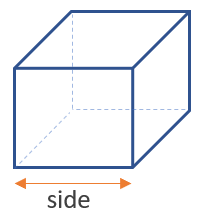The only required information is the side, then you take its cube and you have found the cube's volume. It is the same as multiplying the surface area of one side by the depth of the cube. For this type of figure one barely needs a calculator to do the math.

## Volume of a box

To find the volume of a rectangular box use the formula height x width x length, as seen in the figure below: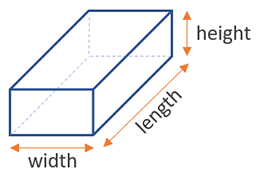To calculate the volume of a box or rectangular tank you need three dimensions: width, length, and height. They are usually easy to measure due to the regularity of the shape. By designating one dimension as the rectangular prism's depth or height, the multiplication of the other two gives us the surface area which then needs to be multiplied by the depth / height to get the volume. To calculate the volume of a tank of a different shape, use our volume of a tank calculator.

## Volume of a cylinder

The volume formula for a cylinder is height x π x (diameter / 2)2, where (diameter / 2) is the radius of the base (d = 2 x r), so another way to write it is height x π x radius2. Visual in the figure below: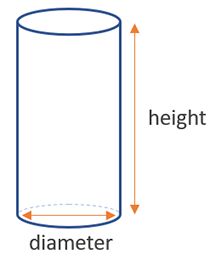You need two measurements: the height of the cylinder and the diameter of its base. In many school formulas the radius is given instead, but in real-world situations it is much easier to measure the diameter instead of trying to pinpoint the midpoint of the circular base so you can measure the radius. Our volume calculator requires that you insert the diameter of the base. Through the diameter the surface area of the base can be calculated and then to get the volume just multiply it by the cylinder's height.

## Volume of a sphere

To find the volume of a sphere, use the formula 4/3 x π x (diameter / 2)3, where (diameter / 2) is the radius of the sphere (d = 2 x r), so another way to write it is 4/3 x π x radius3. Visual on the figure below: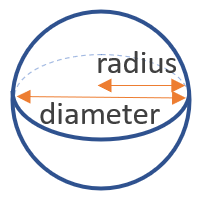Same as a circle, you only need one measurement of the sphere: its diameter or its radius.

## Volume of a cone

The volume formula for a cone is (height x π x (diameter / 2)2) / 3, where (diameter / 2) is the radius of the base (d = 2 x r), so another way to write it is (height x π x radius2) / 3, as seen in the figure below: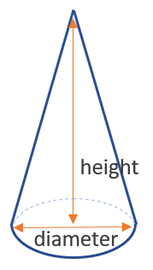Despite being a somewhat complex shape, you only need to know three dimensions to compute the volume of a regular cone. For irregular cones, which are not supported by our tool, yet, you would also need to know the angle of the cone.

## Volume of a triangular prism

The volume formula for a triangular prism is (height x base x length) / 2, as seen in the figure below: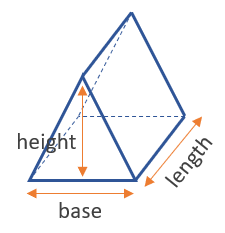Similar to rectangular boxes, you need just three dimensions: height, base, and length in order to find its volume.

## Examples of volume formulae applications

Volume calculations and therefore also formulae have a vast array of practical applications. If you are faced with a construction project, home decoration DIY job, or certain engineering tasks, the calculator will help you if the figure you want to calculate volume of falls within any of the above forms. Complex figures can usually be decomposed, at least approximately, to a sum of the above basic figures.

If you are looking to complete a more specific task, such as to calculate the amount of concrete needed, or the amount of asphalt, gravel, soil, sand, or mulch, it is best to refer to each of these tools respectively.

#### Cite this calculator & page

If you'd like to cite this online calculator resource and information as provided on the page, you can use the following citation:
Georgiev G.Z., "Volume Calculator", [online] Available at: https://www.gigacalculator.com/calculators/volume-calculator.php URL [Accessed Date: 27 Mar, 2023].